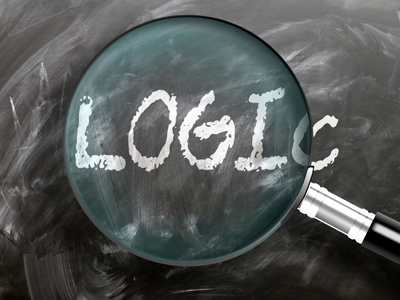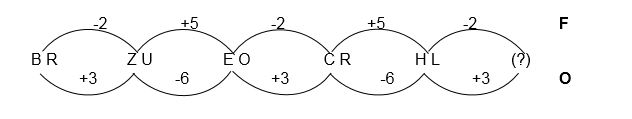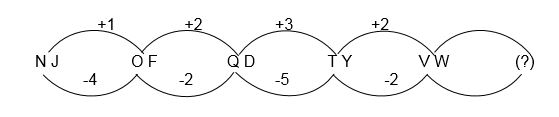You'll need to use logic to solve these types of question.

# VR - Letter Series (2)

As we learned in the previous article, Letter Series (1), Letter Series questions are all about working out through logic, reason and a little maths, the next two letters in a given series.

We have already shown you the most methodical and reliable way to tackle these questions, but sometimes, a different approach is required.

In this article we’ll show you some of the rare occasions when it is better to look at the bigger picture rather than dive straight in and use the usual technique. Although it would work, there is a shortcut.

As ever, let’s show you with some examples.

Example Question One

Find the next letters in the series.

 BD DG GK KP (?)

Looking at the second letter of each pair, it is the same as the first letter of the following pair. The first letter of the answer must therefore be the same as the second letter of the previous pair – it is P. That means we can save time and not worry about working out the first letter of the answer using the counting method.

The second letter of the answer will need to be worked out using the standard method. The second letters of each pair in series are:

 D G K P (?)

The pattern works as follows: if you count on from D you need to move three letters forwards to get to G. Therefore, it is a step of +3. G to K is +4 and K to P is +5. The answer will therefore be P + 6, which works out as V. The overall answer is therefore PV.

Example Question Two

Find the next letters in the series.

 BR ZU EO CR HL (?)

There is no obvious pattern to be found here so we need to use the counting method. Let’s take the first letters of each pair to start with:

 B Z E C H (?)

B to Z seems an odd one to look at – it’s indicative of the key fact that the alphabet can be used as a circular sequence so that if you go back one step from A you go back to Z, while going one step forward from Z will take you to A. If your child doesn’t get the hang of this, write the alphabet on a strip of paper and make it into a ring so that it is an endless loop.

So, B to Z is expressed as -2. In other words, count back two places from B and you reach Z. Then, Z to E is +5, E to C is -2, C to H is +5 so the next in the series will be H-2. The first letter of the answer is therefore F.

The second letter is found in a similar way. Rather than write it all out, here it is expressed using the arcs and numbers:The answer is FO. It is not safe to just look at the first step and assume it will be the same all the way through. The steps alternate and if you want to cut out some time you could look at the fact that we need the SIXTH pair and every even numbered pair has a first letter that’s -2 from the previous, while its second letter is +3 from the one before. In other words, cut out the time spent checking the relation between the fourth and fifth pairs, they won’t tell you anything of any use. The step taken to reach the second pair and the fourth pair is the same, so it should be to reach the sixth pair.

Example Question Three

Find the next letters in the series.

 NJ OF QD TY VW

As there is no simple way to solve this one we need to look to the counting method. You should find it looks like this:This proves that it is necessary to check the relationship between the first five sets of numbers in most cases. If you only looked at the first four pairs, the top line would read +1, +2, +3. We would expect the next in the sequence to be +4 but in fact it’s +2. The final step could be EITHER +5 OR +1, depending on the way you read the series. Either it goes 1, 2, 3, 2, 5, 2, 7, 2, 9 etc. (odd numbered digits increase by 2 each time) OR it’s 1, 2, 3, 2, 1 like a mirror image series.

Similarly, the series for the bottom line could read -4, -2, -5, -2, -6, -2, -7, -2 etc. (odd numbered digits decrease by 1 each time) or -4, -2, -5, -2, -4 like a mirror image series.

Okay, this is all very confusing. Don’t worry. In a fair world and with well-written exam papers you’ll either never get a question this tough OR you’ll have multiple choices to choose from. Tell your child to work out the most sensible answer and check if it’s an option. If not, look for a different answer and see whether that one is!

The most reasonable answer to this question will be AQ but it could also be WS if you use the mirror image series idea. Put simply, encourage your child to use the multiple-choice answers to guide them.

## Sample Tests

So, now that you have learnt exactly how Letter Series work and what the examiners are after, it’s time to get some practise. There are four quizzes on the Education Quizzes site devoted specifically to this type of question.

Try them with your child. See how they get on and use the hints and tips we have learned in this article, and the previous one, to guide them.

You’ll find the quizzes in our Eleven Plus Verbal Reasoning section or, alternatively, you can follow these links:

Letter Series 1

Letter Series 2

Letter Series 3

Letter Series 4

Good luck!